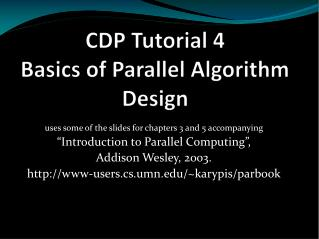DownloadDownload PresentationCDP Tutorial 4 Basics of Parallel Algorithm Design

# CDP Tutorial 4 Basics of Parallel Algorithm Design

Download Presentation## CDP Tutorial 4 Basics of Parallel Algorithm Design

- - - - - - - - - - - - - - - - - - - - - - - - - - - E N D - - - - - - - - - - - - - - - - - - - - - - - - - - -
##### Presentation Transcript

1. CDP Tutorial 4Basics of Parallel Algorithm Design uses some of the slides for chapters 3 and 5 accompanying “Introduction to Parallel Computing”, Addison Wesley, 2003. http://www-users.cs.umn.edu/~karypis/parbook

2. Preliminaries: Decomposition, Tasks, and Dependency Graphs • Parallel algorithm should decompose the problem into tasks that can be executed concurrently. • A decomposition can be illustrated in the form of a directed graph (task dependency graph). • nodes = tasks and edges = dependencies: the result of one task is required for processing the next • Degree of concurrency determines the amount of tasks which can indeed run in parallel. • Critical path is the longest path in the dependency graph – lower bound on program runtime.

3. Example: Multiplying a Dense Matrix with a Vector Observations: While tasks share data (namely, the vector b ), they do not have any control dependencies - i.e., no task needs to wait for the (partial) completion of any other. All tasks are of the same size in terms of number of operations. Is this the maximum number of tasks we could decompose this problem into? Computation of each element of output vector y is independent of other elements. Based on this, a dense matrix-vector product can be decomposed into n independent tasks. Is this the maximum number of tasks we could decompose this problem into?

4. Multiplying a dense matrix with avector – 2n CPUs available Task 2 Task 1 On what kind of platform will we have 2N processors?

6. Granularity of Task Decompositions • The size of tasks into which a problem is decomposed is called granularity

7. Parallelization efficiency • Parallel algorithm scalability factors: • Amdahl’s law: parallel algorithm = parallel part + serial partupper bound on speedup: • Task interaction overhead • CPU utilization • stalls due to data dependencies • imperfect load balancing • Excess computations

8. Guidelines for good parallel algorithm design • Maximize concurrency. • Spread tasks evenly between processors to avoid idling and achieve load balancing. • Execute tasks on critical path as soon as the dependencies are satisfied. • Minimize(overlap) communication between processors.

9. Evaluating parallel algorithm • Speedup • S = Tserial / Tparallel • Efficiency • E = S / #processors • Cost • C = #processors * Tparallel • Scalability • Speedup (efficiency) as a function of number of processors with fixed task size.

10. Simple example: summing n numbers on p CPUs

11. Summing n numbers on p CPUs • Parallel Time = θ((n/p)log p) • Serial Time = θ(n) • Speedup = θ(p/(log p)) • Efficiency = θ(1/log p)

12. Parallel multiplication of 3 matrices • A, B, C – rectangular matrices N x N • We want to compute in parallel: A x B x C • How to achieve the maximum performance?

13. Try 1 2-CPU parallelization: B A TEMP X C TEMP Result X

14. Try 2 3-CPU parallelization: B A C' X C C' Result X Is this a good way?

15. Dynamic task creation - Simple Master-Worker • Identify independent computations A(1,1)*B(1,2) A(1,2)*B(2,2) A(1,1)*B(1,1) A(1,2)*B(2,1) + + T(2,1)*C(2,1) T(1,1)*C(1,1) + Result(1,1) • Create a queue of tasks • Each process picks from the queue and may insert a new task to the queue

16. Hypothetic implementation by multiple threads • Let's assign separate thread for each multiply/sum operation. • How do we coordinate the threads? • Initialize threads to know their dependencies. • Build “producer-consumer” like logic for every thread.